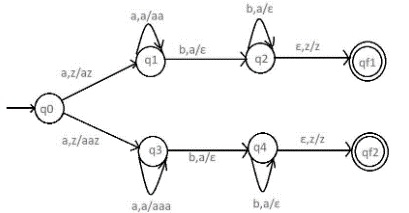# Construct PDA for accepting L = {anb(2n) | n>=1} U {anbn | n>=1}

A push down automata (PDA) can be formally described as seven tuples

(Q, Σ,S, δ,q0,I,F)

Where,

• Q is finite number of states
• Σ is input alphabet
• S is stack symbol
• Δ is the transition function: QX(Σ∪{e})XSXQ
• q0 is the initial state (q0 belongs to Q)
• I is the initial state top symbol
• F is a set of accepting states

## Problem

Construct PDA for L = {anb(2n) | n>=1} ∪ {anbn | n>=1}

## Solution

Let

L = {anb(2n) | n>=1}

{anbn | n>=1}

Construct PDA for L= L1 U L2

So, the strings which are generated by the given language L1 are as follows−

L1={abb,aabbbb,aaabbbbbb,….} and

L2= {ab,aabb,aaabbb,….}

L= L1 U L2 = { abb,aabbbb,aaabbbbbb,….} U {ab,aabb,aaabbb,….}

For language L1

In the language a’s are followed by double the b’s

Whenever ‘a’ comes, push ‘a’ two times in the stack and if ‘a’ comes again then do the same.

When ‘b’ comes then pop one ‘a’ from the stack each time. Note that b comes after ‘a’.

Finally at the end of the strings if nothing is left in the STACK then we can declare that language is accepted in the PDA.

For language L2

For language L2 we have to count equal number of a’s and b’s

This can be achieved by pushing a's in STACK and then we will pop a's whenever "b" comes.

Finally, at the end of the strings if nothing is left in the STACK then we can declare that language is accepted in the PDA.

The PDA for the problem is as follows−## Transition functions

The transition functions are as follows −

Step 1: δ(q0, a, Z) = (q1, aZ)

Step 2: δ(q0, a, a) = (q3, aaz)

Step 3: δδ(q1, a, a) = (q1, aa)

Step 4: δ(q1, b, a) = (q2, ε)

Step 5: δ(q2, b, a) = (q2, ε)

Step 6: δ(q2, ε, Z) = (qf1, Z)

Step 7: δ(q3, a, a) = (q3, aaa)

Step 8: δ(q3, b, a) = (q4, ε)

Step 9: δ(q4, b, a) = (q4, ε)

Step 10: δ(q4, ε, Z) = (qf2, Z)